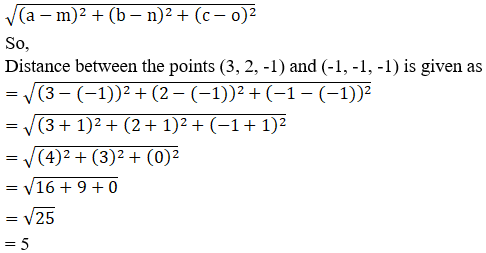Find the distance between the pairs of points A(3, 2, -1) and B (-1, -1, -1)

Asked by Sakshi | 1 year ago |  85

##### Solution :-

A (3, 2, -1) and B (-1, -1, -1)

Given:

The points A (3, 2, -1) and B (-1, -1, -1)

By using the formula,

The distance between any two points (a, b, c) and (m, n, o) is given by,= 5

The Distance between A and B is 5 units.

Answered by Aaryan | 1 year ago

### Related Questions

#### A(1, 2, 3), B(0, 4, 1), C(-1, -1, -3) are the vertices of a triangle ABC. Find the point in which the bisector of the

A(1, 2, 3), B(0, 4, 1), C(-1, -1, -3) are the vertices of a triangle ABC. Find the point in which the bisector of the angle ∠BAC meets BC.

#### The mid-points of the sides of a triangle ABC are given by (-2, 3, 5), (4, -1, 7) and (6, 5, 3). Find the coordinates

The mid-points of the sides of a triangle ABC are given by (-2, 3, 5), (4, -1, 7) and (6, 5, 3). Find the coordinates of A, B and C.

#### If the points A(3, 2, -4), B(9, 8, -10) and C(5, 4, -6) are collinear, find the ratio in which C divided AB.

If the points A(3, 2, -4), B(9, 8, -10) and C(5, 4, -6) are collinear, find the ratio in which C divided AB.